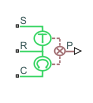# Rotational Power Sensor

Measure average or instantaneous rotational power

•Libraries:
Simscape / Driveline / Sensors

## Description

The Rotational Power Sensor block measures the rotational power change between ports R and C. The physical signal output can be an instantaneous or averaged value depending on what you select for the Measurement type parameter.

When you set:

• Measurement type to ```Instantaneous power```, the instantaneous power is the product of the current angular velocity and torque. Use this setting to analyze peak power transmission and identify heavily loaded components.

• Measurement type to ```Average power over a specified period```, the time-averaged power is the integral of the instantaneous power over one sample period, divided by that period. For simulation times smaller than the sample period, the average power is zero. The power output is constant for each sample period.

• Measurement type to ```Average power over a vibration```, the average vibration power is the integral of the power over one vibration period, divided by that period. The vibration period is the time between two consecutive crossings from negative to positive velocity values. If the vibration has a variable frequency, the averaging period can change. For simulation times smaller than the vibration period, the average power is zero. The power output is constant for each vibration period.

• Measurement type to ```Average power over a revolution```, the average revolution power is the integral of the instantaneous power over one revolution period, divided by that period. The revolution period is defined as the time between two consecutive crossings of zero angular position. If the revolution has a variable frequency, the averaging period can change. For simulation times smaller than the revolution period, the average power is zero. The power output is constant for each revolution period.

## Ports

### Physical Signal

expand all

Physical signal port that outputs the change in rotational power.

### Conserving

expand all

Mechanical rotational conserving port associated with the case.

Mechanical rotational conserving port associated with the load.

Mechanical rotational conserving port associated with driving source.

## Parameters

expand all

Option to measure the instantaneous power or an average power measurement.

• `Instantaneous power` — Output the rotational power measured at the current simulation time step.

• ```Average power over a specified period``` — Output the rotational power averaged over a fixed time period.

• `Average power over a vibration` — Output the rotational power averaged over one vibration period.

• `Average power over a revolution` — Output the rotational power averaged over one revolution period.

Time period that the block uses to compute the average power. For example, you can set this value to the cycle length of a rotating device.

#### Dependencies

To enable this parameter, set Measurement type to ```Average power over a specified period```.

Highest vibration frequency to use to average the rotational power. Very high values can reduce simulation speed. Very low values can result in lower power measurements.

#### Dependencies

To enable this parameter, set Measurement type to `Average power over a vibration`.

Highest speed to use to average the rotational power. Very high values can reduce simulation speed. Very low values can result in lower power measurements.

#### Dependencies

To enable this parameter, set Measurement type to `Average power over a revolution`.

## Version History

Introduced in R2013a